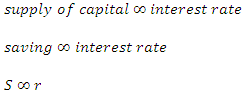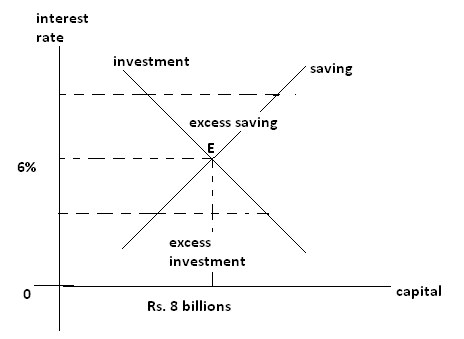# Supply of capital

Supply of capital
It is the amount available for investment. It is called savings. It is the amount left after consumption. It is the monetary value of products left after consumption. It is directly related to interest rate if interest rate is high, the people save more to earn more interest and vice versa.
Symbolically,In the above table, when interest rate is increased from 4% to 6% and 8% supply of capital ( savings) increases from Rs 6 billions to Rs 8 billions and Rs 10 billions respectively. If we represent savings with respect to interest rate, we obtain an upwardly sloped curve.The equilibrium interest rate is given by the point of intersection of investment and savings curves. In the above figures, it is given by point E. However, the actual interest rate may be above or below the equilibrium interest rate. If it is above the level of equilibrium, saving exceeds investment. The excess supply of capital brings the interest rate down to equilibrium level. If it is below the level of equilibrium, investment exceeds savings. The excess demand for capital brigs the interest rate up to equilibrium level. It means sooner or later, the actual interest rate comes to the equilibrium level even it is above or below than it at any instant.

The equilibrium interest rate is given by the point of intersection of investment and savings curves. In the above figures, it is given by point E. However, the actual interest rate may be above or below the equilibrium interest rate. If it is above the level of equilibrium, saving exceeds investment. The excess supply of capital brings the interest rate down to equilibrium level. If it is below the level of equilibrium, investment exceeds savings. The excess demand for capital brigs the interest rate up to equilibrium level. It means sooner or later, the actual interest rate comes to the equilibrium level even it is above or below than it at any instant.

The equilibrium interest rate is given by the point of intersection of investment and savings curves. In the above figures, it is given by point E. However, the actual interest rate may be above or below the equilibrium interest rate. If it is above the level of equilibrium, saving exceeds investment. The excess supply of capital brings the interest rate down to equilibrium level. If it is below the level of equilibrium, investment exceeds savings. The excess demand for capital brigs the interest rate up to equilibrium level. It means sooner or later, the actual interest rate comes to the equilibrium level even it is above or below than it at any instant.

Criticisms

1. Money is not veil and is not just a medium of exchange. It is asset too. More or less money has effect on investment, production, employment level etc.
2. Money is not demanded or borrowed only for investment but also for speculation and precaution
3. This theory is based upon diminishing marginal productivity of capital but there may be increase in marginal productivity of capital due to advancement in technology, improvement in human resource etc.
4. Both demand for capital and supply of capital are not only determined by interest rate but also upon level of income
5. Supply of capital comes not only from saving but also from dishoarding, depreciation fund etc.
6. Saving and investment are not independent of each other. They are affected by each other.